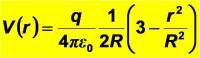# Potential Difference of an Insulating Sphere

TrueStar

## Homework Statement

Charge 6.00 is distributed uniformly over the volume of an insulating sphere that has radius = 5.00 .

What is the potential difference between the center of the sphere and the surface of the sphere?

## Homework Equations

ΔVab= -∫E*dl

Electric field inside an insulating sphere is E=Qr/4∏ε0R3

## The Attempt at a Solution

I am not sure where to begin. I think there is some ingration with the electric field equation, but I don't know if I use the same one for the surface of the sphere.

Homework Helper
You know how the field inside the sphere changes with radius ... you wrote the equation down. So that is a good place to start.

What is the relationship between potential and electric field?

TrueStar
Is it not the first relevant equation I wrote down? The change in potential is the negative integral of the electric field multiplied times the integral of the length...which would be the radius in this case.

TrueStar
I still don't understand how to solve it. I figured out the electric field inside of an insulator however, and why it looks the way it does.

E=Qr/4∏ε0R^3

Where r is the distance of a Gaussian sphere inside the insulator from the center to r.

However, how would I know what r is, and does it ultimately matter? I also still don't see how this will fit with the ΔV equation, assuming that is what I need. I thought about considering the center of the sphere as a point charge, but that didn't get me the right answer.

Last edited:
Homework Helper
me said:
What is the relationship between potential and electric field?
Is it not the first relevant equation I wrote down?
No - I mean you need the solution to the relation for the inside of a sphere.

If you haven't seen it before in your course then, yes, you will have t do the integration (if you've seen the differential form of Maxwell'd equations - that's easier.) Which also means that I cannot tell you how to do it ... you have to make the attempt and then I can help you out where you get stuck.

how would I know what r is
Two values of r are given to you.
I also still don't see how this will fit with the ΔV equation
You need an equation for V(r), then you can find the potential at different radii, and, therefore, the difference in potential for any two radii.
I thought about considering the center of the sphere as a point charge, but that didn't get me the right answer.
Well no. The charge is the charge-density times the volume and the volume of a point is zero - so the total charge at the point r=0 is zero.

Note: below, under "similar threads" there are a lot of clues.

TrueStar
I looked at the other similar threads earlier today and one of them seemed to be stuck in the same place I am. Like the poster, I think I was just missing part of the equation...

V(r)-V(R)=∫E*dl

Plug in everything and solve for V(r) which turns out to look like this.I tried to figure out how to get this and I can't. I am not even sure if this will be a given equation on the upcoming midterm. My professor says he will provide us with an equation sheet, but he has yet to upload it.

But I suspect you need to revise electrostatic potentials from the beginning - and study the examples already provided. If you want you can try $$V(r)=-\int_\infty^r E(r^\prime)dr^\prime$$... from knowing E(r).